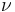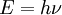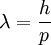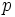# Planck's constant

(Redirected from Planck's Constant)

Planck's constant, denoted, is a fundamental constant in physics. The current value of Planck's constant isJoule-seconds (numbers in brackets represent uncertainty in the last two decimal places).

## History

It was first used by Max Planck to explain the radiation curves of black-bodies. He supposed that light could be modelled as a series of particles (now called photons), each with an energy proportional to its frequnecy,. The constant of proportionality was Planck's constant. Mathematically this can be expressed as:In 1924, Louis De Broglie proposed Wave-particle duality, the idea that matter (protons and electrons for example) had a wave-like nature. Here too, is Planck's constant:whereis the wavelength of the particleis the momentum of the particle

The Planck constant also occurs in equations fundamental to quantum mechanics, such as the Schrodinger equation.

## Reduced Planck's Constant

Planck's constant often occurs in equations with the mathematical constant. As such, a factor ofmay be taken into Planck's constant asThe resulting constant is pronounced "h-bar".